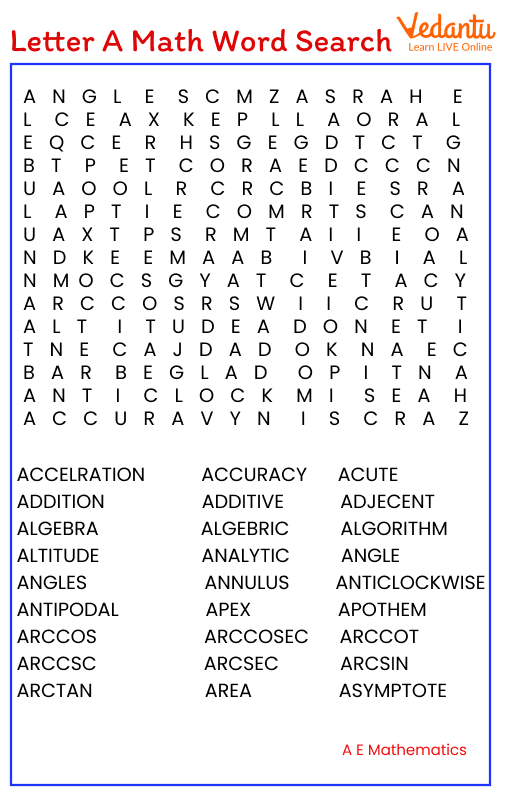Courses
Courses for Kids
Free study material
Free LIVE classes
MoreLIVE
Join Vedantu’s FREE Mastercalss

There are numerous words in mathematics beginning with the letter A. Remembering these words and terms together can help kids refrain from forgetting or mixing up the meanings of these words. This can happen if these words are not revised or retained separately. Let us study the meanings of some of these words in mathematics that start with the letter A.Some mathematical words starting with A

## List of 10 Maths Words Starting with the Letter A

The following is a list of mathematical words starting with the letter A. Study and learn them thoroughly to be able to use them at ease in solving mathematical problems.

1. Algorithm - An algorithm can be defined as a step-by-step process to solve a given problem or to navigate through a situation.

2. Arc - An Arc is a part of the curved circumference of a circle.

3. Addend - Addends are the numbers or components in maths that are to be added to obtain a sum. For instance, in the addition 7 + 8 + 5, the numbers 7, 8, and 5 are the addends.

4. Acute Angle - An angle measuring less than 90 degrees is known as an acute angle. For instance, an angle measuring 75 degrees is an acute angle.

5. Area - Area is the 2-dimensional space covered by a boundary. Area is measured in square units and is a general measure of the amount of surface occupied by lines, curves, or boundaries.

6. Axis - An axis is a constant reference line used to measure the coordinates in a coordinate system. In a 2D coordinate system, the horizontal axis is known as the x-axis and the vertical axis is called the y-axis.

7. Arithmetric - The branch of mathematics dealing with the study of numbers with various operations being used on them is called Arithmetic. Some of the basic operations in maths are addition, subtraction, multiplication, and division.

8. Algebra - The branch of mathematics that helps to represent problems or situations in the form of specific or generalised mathematical expressions is known as Algebra.

9. Array - An array is a collection of numbers or objects that have been arranged in rows and columns.

10. Ascending Order - ascending order of arrangement implies that numbers or objects have been arranged in the increasing order, that is, from the smallest to the largest.

## Conclusion

The words starting with the letter A that have been discussed in this article are extremely important in mathematics and must be remembered. Since they all begin with A, it will be easy to memorise them together. But it can also be easy to confuse some of these words and should therefore be studied distinctly as well.

Last updated date: 22nd Sep 2023
Total views: 138.3k
Views today: 4.38k